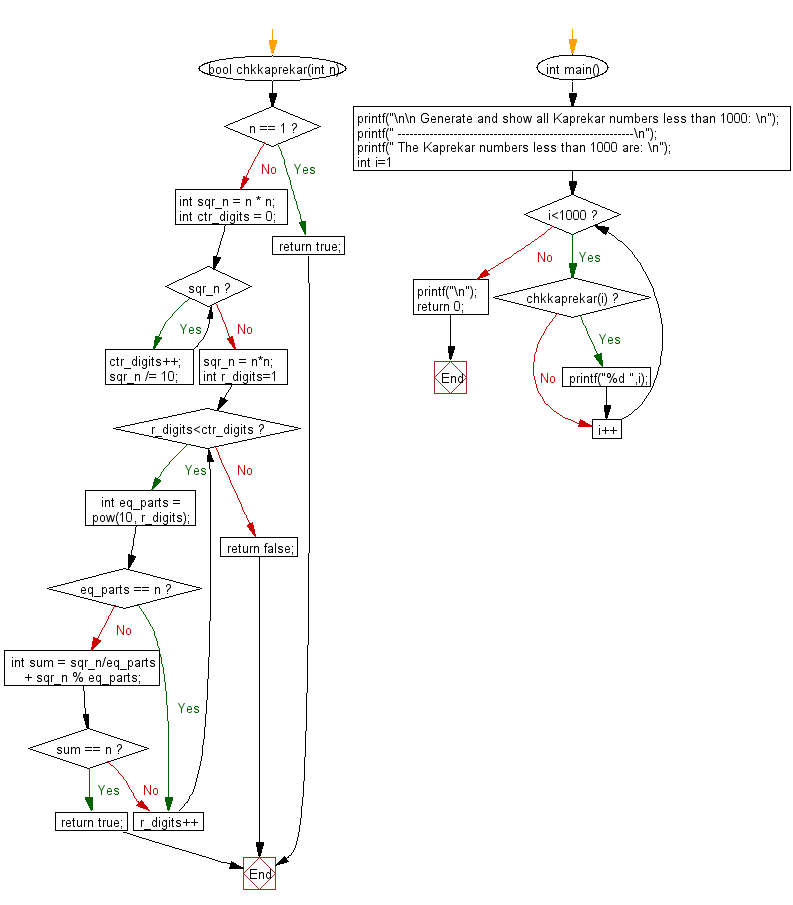﻿ C Program: Show all Kaprekar numbers less than 1000# C Exercises: Generate and show all Kaprekar numbers less than 1000

## C Numbers: Exercise-7 with Solution

Write a program in C to generate and show all Kaprekar numbers less than 1000.

Sample Solution:

C Code:

``````# include <stdio.h>
# include <stdbool.h>
# include <math.h>

bool chkkaprekar(int n)
{
if (n == 1)
return true;

int sqr_n = n * n;
int ctr_digits = 0;
while (sqr_n)
{
ctr_digits++;
sqr_n /= 10;
}

sqr_n = n*n;

for (int r_digits=1; r_digits<ctr_digits; r_digits++)
{
int eq_parts = pow(10, r_digits);

if (eq_parts == n)
continue;

int sum = sqr_n/eq_parts + sqr_n % eq_parts;
if (sum == n)
return true;
}
return false;
}
int main()
{
printf("\n\n Generate and show all Kaprekar numbers less than 1000: \n");
printf(" -----------------------------------------------------------\n");
printf(" The Kaprekar numbers less than 1000 are: \n");
for (int i=1; i<1000; i++)
{
if (chkkaprekar(i))
{
printf("%d ",i);

}

}
printf("\n");
return 0;
}
```
```

Sample Output:

``` The Kaprekar numbers less than 1000 are:
1 9 45 55 99 297 703 999
```

Pictorial Presentation:Flowchart:C Programming Code Editor:

What is the difficulty level of this exercise?

Test your Programming skills with w3resource's quiz.

﻿

## C Programming: Tips of the Day

Why do C and C++ compilers allow array lengths in function signatures when they're never enforced?

It is a quirk of the syntax for passing arrays to functions.

Actually it is not possible to pass an array in C. If you write syntax that looks like it should pass the array, what actually happens is that a pointer to the first element of the array is passed instead.

Since the pointer does not include any length information, the contents of your [] in the function formal parameter list are actually ignored.

Ref : https://bit.ly/3fhlvdH